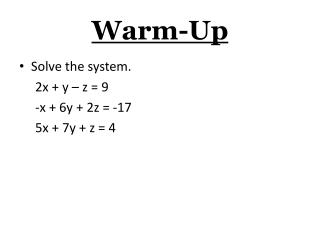DownloadDownload PresentationWarm-Up

# Warm-Up

Download Presentation## Warm-Up

- - - - - - - - - - - - - - - - - - - - - - - - - - - E N D - - - - - - - - - - - - - - - - - - - - - - - - - - -
##### Presentation Transcript

1. Warm-Up • Solve the system. 2x + y – z = 9 -x + 6y + 2z = -17 5x + 7y + z = 4

2. Section 3-4 (DAY 2) Solve Systems of Linear Equations in Three Variables Using a Calculator

3. Steps for Solving • Solve (using a Calculator) -x +5y – z = -16 2x + 3y + 4z = 18 x + y – z = -8 • Create the following matrix (table)

4. Push 2nd x-1 (TI -83 push the Matrix) Arrow over to edit Push enter Put in the number of rows and the number of columns (for this example 3 enter 4 enter) Type in the numbers pushing enter after each one. Push 2nd mode (the matrix has been saved)

5. Push 2nd x-1 (TI -83 push matrix) Arrow over to math Arrow down to rref( [Letter B in List] Push enter Push 2nd x-1 (TI -83 push matrix) Enter the matrix letter where your matrix is (This example it will be in A) Close the ( ) Push enter

6. Solution: (0, -2, 6) Which is: x = 0 y = -2 z = 6 The last column is your solution in x, y, z order.

7. Homework Section 3-4 (DAY 2) Pages 182 – 185 (23, 25, 26, 38, 44, 46)